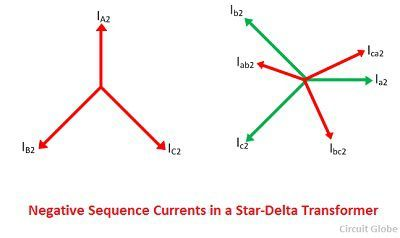# Phase Shift in Star Delta Transformer

The phase shift is the angular displacement between the maximum positive value of the alternating quantities having the same frequency. In other words, the angular displacement between high voltage and the low voltage terminal and the corresponding neutral points (real or imaginary), expressed with reference to high voltage side is known as phase displacement (or shift) of the transformer.

There is no phase shift between the star-star and delta-delta 3-phase transformer. Most of the power transformer is either star-delta or delta star connected. In such type of transformers, even in the normal operating condition, the phase-to-phase voltages and phase-to-neutral voltage of high voltage side are displaced from the corresponding voltage of low voltage side. Similarly the current on the two sides are displaced.

Considered 3-phase star-delta transformer with primary side Y-connected and secondary with delta-connected as shown in the figure below. The polarity markings are indicated on each phase. The dots on the windings indicate the terminals which are positive at the same time on the undotted terminals.The phases on the star sides are marked as A, B, C and the phases on the delta side are marked as a, b, c. The labelling is indicated to the diagram corresponding to + 90º connections in which the positive sequences on the delta side is lead by 90º corresponding to star side. Thus, the lines currents flow through the phase a and A.

The alternating way is to label delta as b→a, c→b and a→c; Thus we get a standard yd1, -30º connection. If the polarities on the delta side are also reversed, we have standard yd11, 30º connection. The yd11 are used for line-to-line voltage, and yd1 are used for line currents and phase (line-to-neutral) voltage. Line-to-line transformation ratio is being taken to be unity.

The positive and negative sequence voltages on the primary (star) and secondary (delta) sides of the transformer are shown below. When the power flow is reversed, and the delta of the transformer acts as primary and star act as secondary, then the voltage phasor does not change, but all the current phasor are reverse.Thus, the magnitude of phase shift is same for positive and negative sequence component. The direction of phase shift is in the case of negative phase sequence is the reverse of that applies to the positive phase sequence component (due to reverse phase sequence).The magnitude and direction of phase shift depend on the transformer group and allocation of phase references. The phase shift of zero sequence quantities needs not to be considered in star-delta transformer because the zero sequence currents do not flow in lines on the delta-connected side.

### 2 thoughts on “Phase Shift in Star Delta Transformer”

1. yes because the same vector group transformer is connected in parallel only otherwise phase shift will be changed that becomes effect on circulating current.